International
Tables for
Crystallography
Volume B
Reciprocal space
Edited by U. Shmueli

International Tables for Crystallography (2010). Vol. B, ch. 1.3, pp. 78-79   | 1 | 2 |

## Section 1.3.4.3.3. Interaction between symmetry and decomposition

G. Bricognea

aGlobal Phasing Ltd, Sheraton House, Suites 14–16, Castle Park, Cambridge CB3 0AX, England, and LURE, Bâtiment 209D, Université Paris-Sud, 91405 Orsay, France

#### 1.3.4.3.3. Interaction between symmetry and decomposition

| top | pdf |

Suppose that the space-group action is reducible, i.e. that for each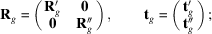by Schur's lemma, the decimation matrix must then be of the formif it is to commute with all the.

Putting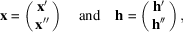we may defineand write(direct sum) as a shorthand for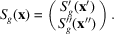We may also define the representation operatorsandacting on functions ofand, respectively (as in Section 1.3.4.2.2.4), and the operatorsandacting on functions ofand, respectively (as in Section 1.3.4.2.2.5). Then we may writeandin the sense that g acts onbyand onbyThus equipped we may now derive concisely a general identity describing the symmetry properties of intermediate quantities of the form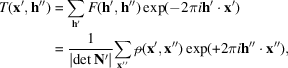which arise through partial transformation of F onor ofon. The action ofon these quantities will be

 (i) throughon the function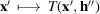, (ii) throughon the function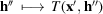,

and hence the symmetry properties of T are expressed by the identityApplying this relation not to T but togivesi.e.If the uniquewere initially indexed by(see Section 1.3.4.2.2.2), this formula allows the reindexing of the intermediate resultsfrom the initial formto the final formon which the second transform (on) may now be performed, giving the final resultsindexed bywhich is an asymmetric unit. An analogous interpretation holds if one is going fromto F.

The above formula solves the general problem of transposing from one invariant subspace to another, and is the main device for decomposing the CDFT. Particular instances of this formula were derived and used by Ten Eyck (1973); it is useful for orthorhombic groups, and for dihedral groups containing screw axeswith g.c.d.. For comparison with later uses of orbit exchange, it should be noted that the type of intermediate results just dealt with is obtained after transforming on all factors in one summand.

A central piece of information for driving such a decomposition is the definition of the full asymmetric unit in terms of the asymmetric units in the invariant subspaces. As indicated at the end of Section 1.3.4.2.2.2, this is straightforward when G acts without fixed points, but becomes more involved if fixed points do exist. To this day, no systematic `calculus of asymmetric units' exists which can automatically generate a complete description of the asymmetric unit of an arbitrary space group in a form suitable for directing the orbit exchange process, although Shenefelt (1988)has outlined a procedure for dealing with space group P622 and its subgroups. The asymmetric unit definitions given in Volume A of International Tables are incomplete in this respect, in that they do not specify the possible residual symmetries which may exist on the boundaries of the domains.

### References

Shenefelt, M. (1988). Group Invariant Finite Fourier Transforms. PhD thesis, Graduate Centre of the City University of New York.
Ten Eyck, L. F. (1973). Crystallographic fast Fourier transforms. Acta Cryst. A29, 183–191.